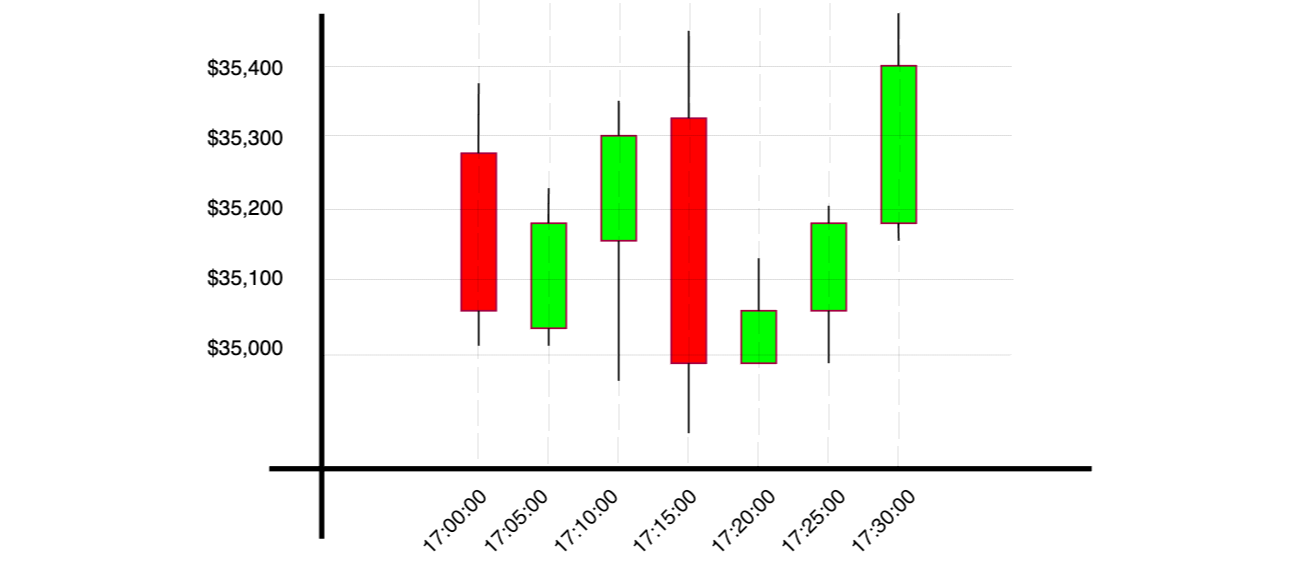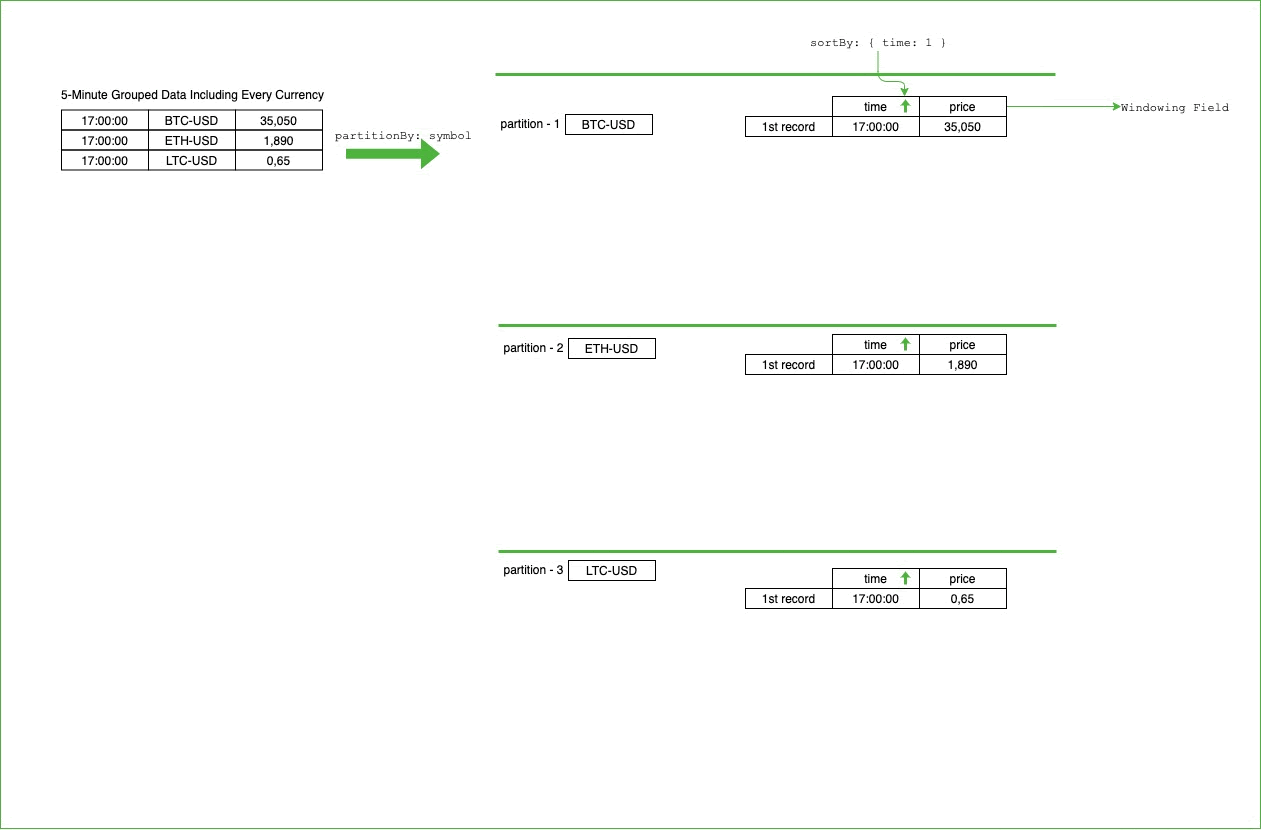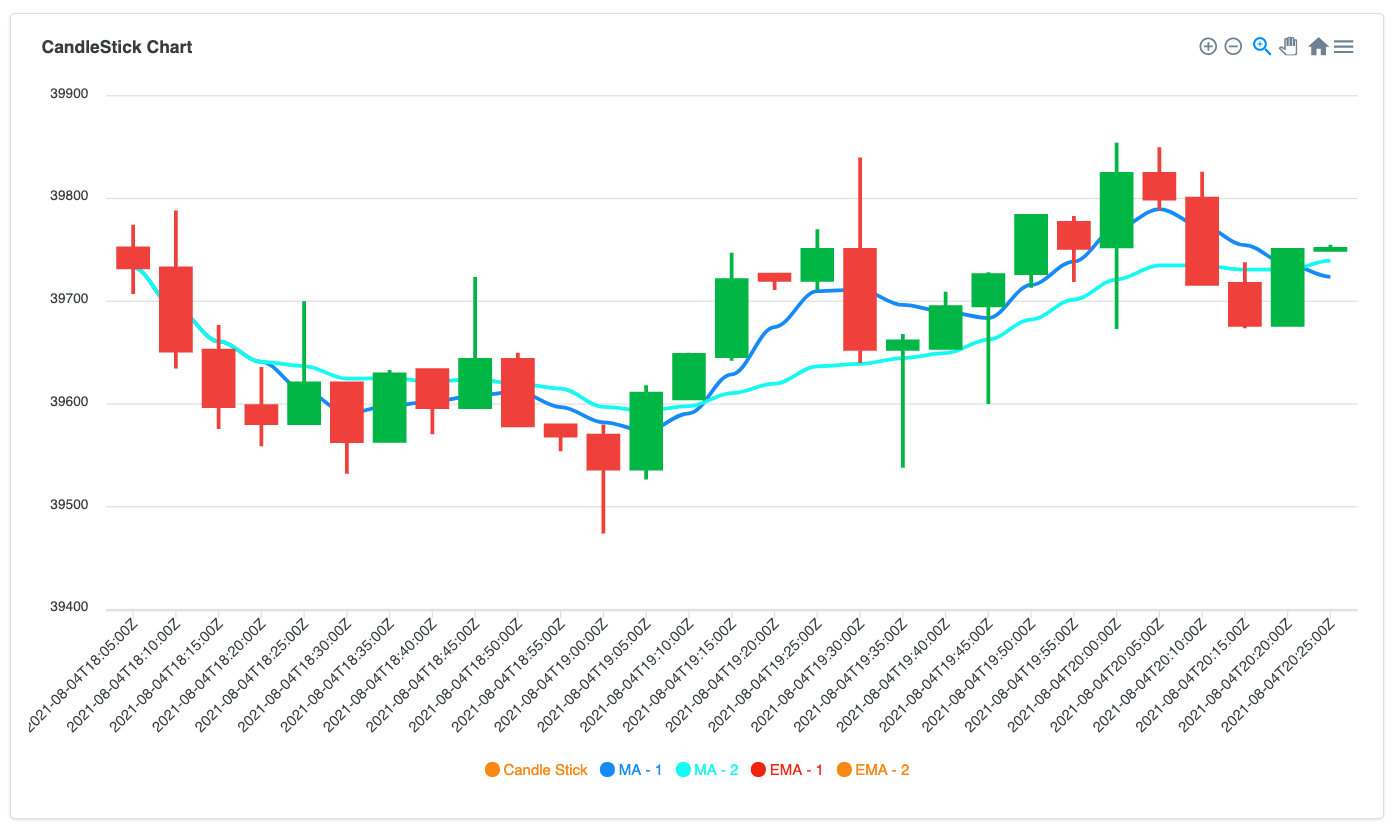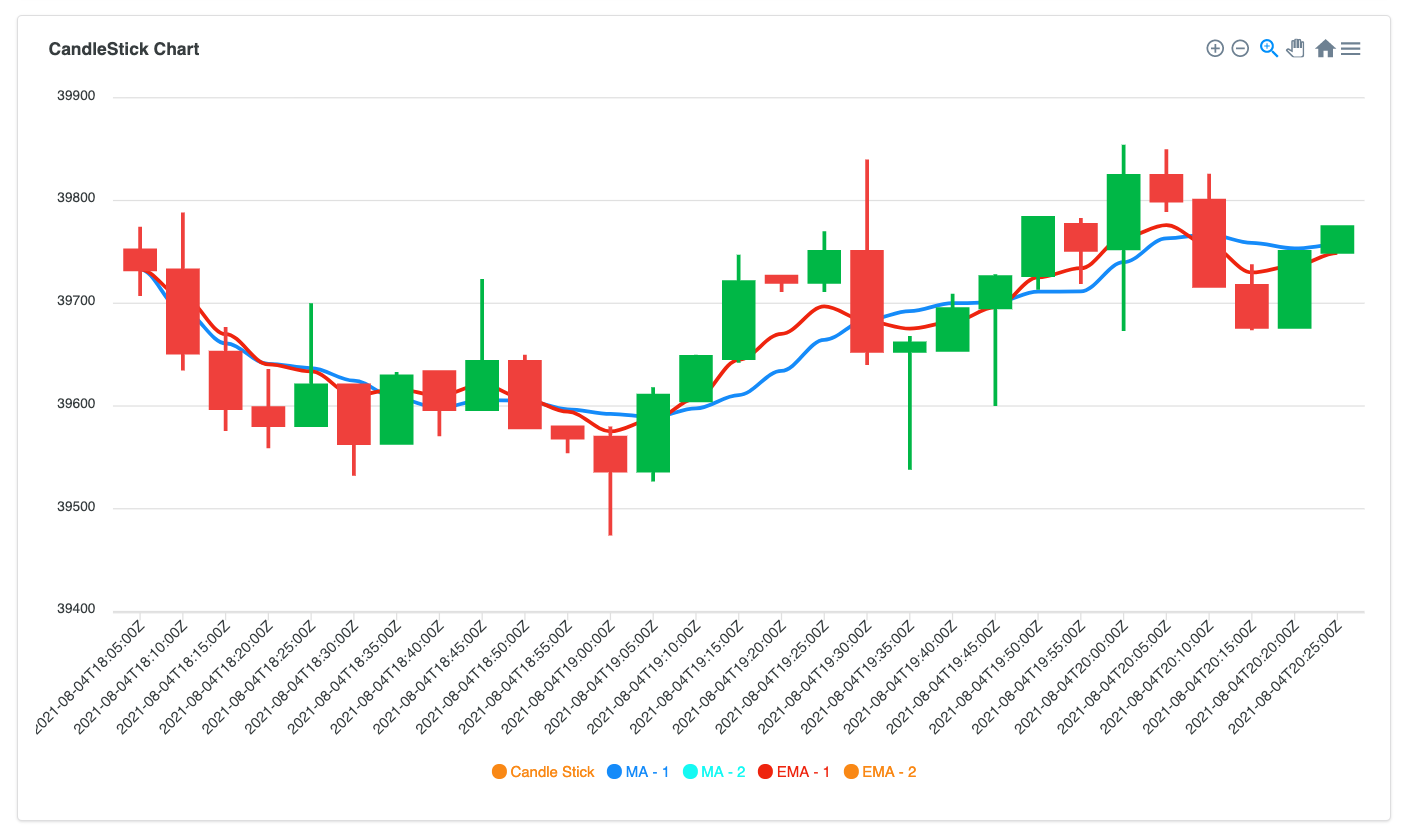HomeLearnArticleCurrency Analysis with Time Series Collections #2 — Simple Moving Average and Exponential Moving Average Calculation

Currency Analysis with Time Series Collections #2 — Simple Moving Average and Exponential Moving Average Calculation

Updated: Aug 27, 2021 |

Published: Aug 27, 2021

• MongoDB
• JavaScript
• Time Series

By Fuat Sungur

In the previous post, we learned how to group currency data based on given time intervals to generate candlestick charts to perform trend analysis. In this article, we’ll learn how the moving average can be calculated on time-series data.

Moving average is a well-known financial technical indicator that is commonly used either alone or in combination with other indicators. Additionally, the moving average is included as a parameter of other financial technical indicators like MACD. The main reason for using this indicator is to smooth out the price updates to reflect recent price changes accordingly. There are many types of moving averages but here we’ll focus on two of them: Simple Moving Average (SMA) and Exponential Moving Average (EMA).

#Simple Moving Average (SMA)

This is the average price value of a currency/stock within a given period.

Let’s calculate the SMA for the BTC-USD currency over the last three data intervals, including the current data. Remember that each stick in the candlestick chart represents five-minute intervals. Therefore, for every interval, we would look for the previous three intervals.

First we’ll group the BTC-USD currency data for five-minute intervals:

 1 db.ticker.aggregate([ 2 { 3 \$match: { 4 symbol: "BTC-USD", 5 }, 6 }, 7 { 8 \$group: { 9 _id: { 10 symbol: "\$symbol", 11 time: { 12 \$dateTrunc: { 13 date: "\$time", 14 unit: "minute", 15 binSize: 5 16 }, 17 }, 18 }, 19 high: { \$max: "\$price" }, 20 low: { \$min: "\$price" }, 21 open: { \$first: "\$price" }, 22 close: { \$last: "\$price" }, 23 }, 24 }, 25 { 26 \$sort: { 27 "_id.time": 1, 28 }, 29 }, 30 ]);

And, we will have the following candlestick chart:We have four metrics for each interval and we will choose the close price as the numeric value for our moving average calculation. We are only interested in _id (a nested field that includes the symbol and time information) and the close price. Therefore, since we are not interested in high, low, open prices for SMA calculation, we will exclude it from the aggregation pipeline with the \$project aggregation stage:

 1 { 2 \$project: { 3 _id: 1, 4 price: "\$close", 5 }, 6 }

After we grouped and trimmed, we will have the following dataset:

 1 {"_id": {"time": ISODate("20210101T17:00:00"), "symbol" : "BTC-USD"}, "price": 35050} 2 {"_id": {"time": ISODate("20210101T17:05:00"), "symbol" : "BTC-USD"}, "price": 35170} 3 {"_id": {"time": ISODate("20210101T17:10:00"), "symbol" : "BTC-USD"}, "price": 35280} 4 {"_id": {"time": ISODate("20210101T17:15:00"), "symbol" : "BTC-USD"}, "price": 34910} 5 {"_id": {"time": ISODate("20210101T17:20:00"), "symbol" : "BTC-USD"}, "price": 35060} 6 {"_id": {"time": ISODate("20210101T17:25:00"), "symbol" : "BTC-USD"}, "price": 35150} 7 {"_id": {"time": ISODate("20210101T17:30:00"), "symbol" : "BTC-USD"}, "price": 35350}

Once we have the above dataset, we want to enrich our data with the simple moving average indicator as shown below. Every interval in every symbol will have one more field (sma) to represent the SMA indicator by including the current and last three intervals:

 1 {"_id": {"time": ISODate("20210101T17:00:00"), "symbol" : "BTC-USD"}, "price": 35050, "sma": ?} 2 {"_id": {"time": ISODate("20210101T17:05:00"), "symbol" : "BTC-USD"}, "price": 35170, "sma": ?} 3 {"_id": {"time": ISODate("20210101T17:10:00"), "symbol" : "BTC-USD"}, "price": 35280, "sma": ?} 4 {"_id": {"time": ISODate("20210101T17:15:00"), "symbol" : "BTC-USD"}, "price": 34910, "sma": ?} 5 {"_id": {"time": ISODate("20210101T17:20:00"), "symbol" : "BTC-USD"}, "price": 35060, "sma": ?} 6 {"_id": {"time": ISODate("20210101T17:25:00"), "symbol" : "BTC-USD"}, "price": 35150, "sma": ?} 7 {"_id": {"time": ISODate("20210101T17:30:00"), "symbol" : "BTC-USD"}, "price": 35350, "sma": ?}

How is it calculated? For the time, 17:00:00, the calculation of SMA is very simple. Since we don’t have the three previous data points, we can take the existing price (35050) at that time as average. If we don’t have three previous data points, we can get all the available possible price information and divide by the number of price data.

The harder part comes when we have more than three previous data points. If we have more than three previous data points, we need to remove the older ones. And, we have to keep doing this as we have more data for a single symbol. Therefore, we will calculate the average by considering only up to three previous data points. The below table represents the calculation step by step for every interval:

TimeSMA Calculation for the window (3 previous + current data points)
17:00:0035050/1
17:05:00(35050+35170)/2
17:10:00(35050+35170+35280)/3
17:15:00(35050+35170+35280+34910)/4
17:20:00(35170+35280+34910+35060)/4
*oldest price data (35050) discarded from the calculation
17:25:00(35280+34910+35060+35150)/4
*oldest price data (35170) discarded from the calculation
17:30:00(34190+35060+35150+35350)/4
*oldest price data (35280) discarded from the calculation

As you see above, the window for the average calculation is moving as we have more data.

#Window Functions

Until now, we learned the theory of moving average calculation. How can we use MongoDB to do this calculation for all of the currencies?

MongoDB 5.0 introduced a new aggregation stage, \$setWindowFields, to perform operations on a specified range of documents (window) in the defined partitions. Because it also supports average calculation on a window through \$avg operator, we can easily use it to calculate Simple Moving Average:

 1 { 2 \$setWindowFields: { 3 partitionBy: "_id.symbol", 4 sortBy: { "_id.time": 1 }, 5 output: { 6 sma: { 7 \$avg: "\$price", 8 window: { documents: [-3, 0] }, 9 }, 10 }, 11 }, 12 }

We chose the symbol field as partition key. For every currency, we have a partition, and each partition will have its own window to process that specific currency data. Therefore, when we’d like to process sequential data of a single currency, we will not mingle the other currency’s data.

After we set the partition field, we apply sorting to process the data in an ordered way. The partition field provides processing of single currency data together. However, we want to process data as ordered by time. As we see in how SMA is calculated on the paper, the order of the data matters and therefore, we need to specify the field for ordering.

After partitions are set and sorted, then we can process the data for each partition. We generate one more field, “sma”, and we define the calculation method of this derived field. Here we set three things:

• The operator that is going to be executed (\$avg).
• The field (\$price) where the operator is going to be executed on.
• The boundaries of the window ([-3,0]).
• [-3: “start from 3 previous data points”.
• 0]: “end up with including current data point”.

• We can also set the second parameter of the window as “current” to include the current data point rather than giving numeric value.

Moving the window on the partitioned and sorted data will look like the following. For every symbol, we’ll have a partition, and all the records belonging to that partition will be sorted by the time information:Then we will have the sma field calculated for every document in the input stream. You can apply \$round operator to trim to the specified decimal place in a \$set aggregation stage:

 1 { 2 \$set: { 3 sma: { \$round: ["\$sma", 2] }, 4 }, 5 }

If we bring all the aggregation stages together, we will end-up with this aggregation pipeline:

 1 db.ticker.aggregate([ 2 { 3 \$match: { 4 symbol: "BTC-USD", 5 }, 6 }, 7 { 8 \$group: { 9 _id: { 10 symbol: "\$symbol", 11 time: { 12 \$dateTrunc: { 13 date: "\$time", 14 unit: "minute", 15 binSize: 5, 16 }, 17 }, 18 }, 19 high: { \$max: "\$price" }, 20 low: { \$min: "\$price" }, 21 open: { \$first: "\$price" }, 22 close: { \$last: "\$price" }, 23 }, 24 }, 25 { 26 \$sort: { 27 "_id.time": 1, 28 }, 29 }, 30 { 31 \$project: { 32 _id: 1, 33 price: "\$close", 34 }, 35 }, 36 { 37 \$setWindowFields: { 38 partitionBy: "_id.symbol", 39 sortBy: { "_id.time": 1 }, 40 output: { 41 sma: { 42 \$avg: "\$price", 43 window: { documents: [-3, 0] }, 44 }, 45 }, 46 }, 47 }, 48 { 49 \$set: { 50 sma: { \$round: ["\$sma", 2] }, 51 }, 52 }, 53 ]);

You may want to add more calculated fields with different options. For example, you can have two SMA calculations with different parameters. One of them could include the last three points as we have done already, and the other one could include the last 10 points, and you may want to compare both. Find the query below:

 1 { 2 \$setWindowFields: { 3 partitionBy: "_id.symbol", 4 sortBy: { "_id.time": 1 }, 5 output: { 6 sma_3: { 7 \$avg: "\$price", 8 window: { documents: [-3, 0] }, 9 }, 10 sma_10: { 11 \$avg: "\$price", 12 window: { documents: [-10, 0] }, 13 }, 14 }, 15 }, 16 }

Here in the above code, we set two derived fields. The sma_3 field represents the moving average for the last three data points, and the sma_10 field represents the moving average for the 10 last data points. Furthermore, you can compare these two moving averages to take a position on the currency or use it for a parameter for your own technical indicator.

The below chart shows two moving average calculations. The line with blue color represents the simple moving average with the window [-3,0]. The line with the turquoise color represents the simple moving average with the window [-10,0]. As you can see, when the window is bigger, reaction to price change gets slower:You can even enrich it further with the additional operations such as covariance, standard deviation, and so on. Check the full supported options here. We will cover the Exponential Moving Average here as an additional operation.

#Exponential Moving Average (EMA)

EMA is a kind of moving average. However, it weighs the recent data higher. In the calculation of the Simple Moving Average, we equally weight all the input parameters. However, in the Exponential Moving Average, based on the given parameter, recent data gets more important. Therefore, Exponential Moving Average reacts faster than Simple Moving Average to recent price updates within the similar size window.

\$expMovingAvg has been introduced in MongoDB 5.0. It takes two parameters: the field name that includes numeric value for the calculation, and N or alpha value. We’ll set the parameter N to specify how many previous data points need to be evaluated while calculating the moving average and therefore, recent records within the N data points will have more weight than the older data. You can refer to the documentation for more information:

 1 { 2 \$expMovingAvg: { 3 input: "\$price", 4 N: 5 5 } 6 }

In the below diagram, SMA is represented with the blue line and EMA is represented with the red line, and both are calculated by five recent data points. You can see how the Simple Moving Average reacts slower to the recent price updates than the Exponential Moving Average even though they both have the same records in the calculation:#Conclusion

MongoDB 5.0, with the introduction of Windowing Function, makes calculations much easier over a window. There are many aggregation operators that can be executed over a window, and we have seen \$avg and \$expMovingAvg in this article.

Here in the given examples, we set the window boundaries by including the positional documents. In other words, we start to include documents from three previous data points to current data point (documents: [-3,0]). You can also set a range of documents rather than defining position.

For example, if the window is sorted by time, you can include the last 30 minutes of data (whatever number of documents you have) by specifying the range option as follows: range: [-30,0], unit: "minute". Now, we may have hundreds of documents in the window but we know that we only include the documents that are not older than 30 minutes than the current data.

You can also materialize the query output into another collection through \$out or \$merge aggregation stages. And furthermore, you can enable change streams or Database Triggers on the materialized view to automatically trigger buy/sell actions based on the result of technical indicator changes.

More from this series

Currency Analysis with MongoDB 5.0 Time Series Collections
• Currency Analysis with Time Series Collections #1 — Generating Candlestick Charts Data
• Currency Analysis with Time Series Collections #2 — Simple Moving Average and Exponential Moving Average Calculation
• Currency Analysis with Time Series Collections #3 — MACD and RSI Calculation